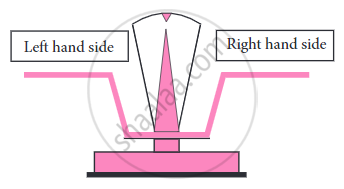# Balancing an Equation

## Notes

### Solving an Equation:

• A balanced equation is like a weighing balance with equal weights in the two pans.

• An equation is like a weighing balance with equal weights on both its pans, in which case the arm of the balance is exactly horizontal. If we add the same weights to both the pans, the arm remains horizontal. Similarly, if we remove the same weights from both the pans, the arm remains horizontal. On the other hand, if we add different weights to the pans or remove different weights from them, the balance is tilted; that is, the arm of the balance does not remain horizontal.

• Thus if we fail to do the same mathematical operation with the same number on both sides of an equality, the equality may not hold.• The value of the variable which balances or satisfies the equation is called the ‘solution’ to the equation.

• To solve an equation is to find the value of the variable in the equation or to find the solution to the equation.

• Example, The solution to the equation ‘x + 190 = 300’ is 110.

## Mathematical Operations on Expressions:

• If the same operation is carried out on both sides of an equation every time, the equation remains balanced. When any of the following operations are carried out on an equation, the equation remains balanced.

1. Adding the same number to both sides:
Let us now add 8 to both sides; as a result
LHS = 8 – 3 + 8 = 5 + 8 = 13
RHS = 4 + 1 + 8 = 5 + 8 = 13.

2. Subtracting the same number from both sides:
Let us now subtract 3 from both the sides; as a result,
LHS = 8 – 3 – 3 = 5 – 3 = 2
RHS = 4 + 1 – 3 = 5 – 3 = 2.

3. Multiplying both sides by the same number:
Let us multiply both the sides of the equality by 3, we get
LHS = 3 × (8 – 3) = 3 × 5 = 15,
RHS = 3 × (4 + 1) = 3 × 5 = 15.

4. Dividing both sides by the same non-zero number:
Let us now divide both sides of the equality by 2.
LHS = (8 – 3) ÷ 2 = 5 ÷ 2 = 5/2
RHS = (4 + 1) ÷ 2 = 5 ÷ 2 = 5/2.

5. Exchanging the two sides:
Let us now exchange the following two sides.
5 m + 7 = 17
5 m = 17 – 7
5 m = 10
m = 10/5
m = 2.

If you would like to contribute notes or other learning material, please submit them using the button below.

### Shaalaa.com

Solving Equations & Transposition Method [00:22:14]
S
0%